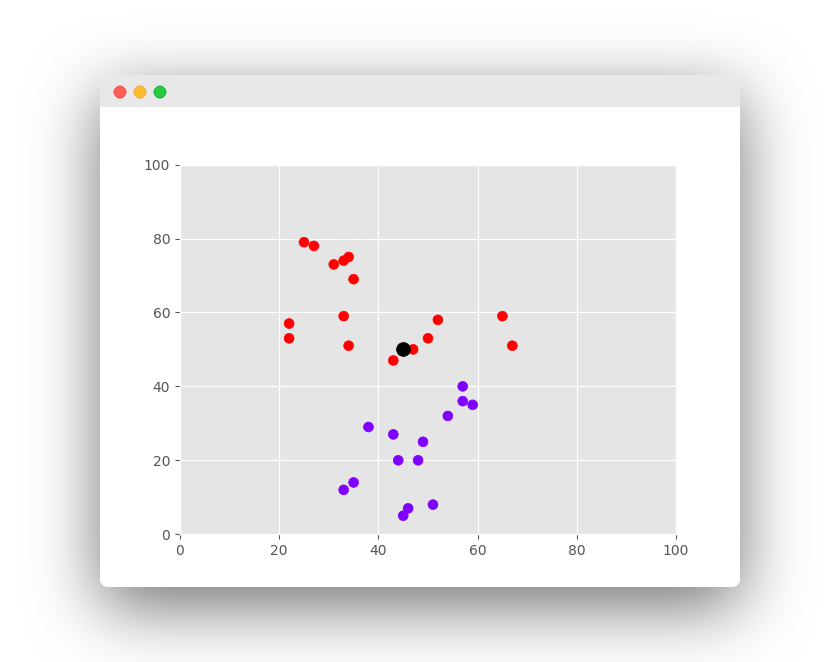Meet K-Nearest Neighbors, one of the simplest Machine Learning Algorithms.

This algorithm is used for Classification and Regression. In both uses, the input consists of the k closest training examples in the feature space. On the other hand, the output depends on the case.

• In K-Nearest Neighbors Classification the output is a class membership.
• In K-Nearest Neighbors Regression the output is the property value for the object.

K-Nearest Neighbors is easy to implement and capable of complex classification tasks.

Related course: Python Machine Learning Course

## knn

### k-nearest neighbors

It is called a lazy learning algorithm because it doesn’t have a specialized training phase.

It doesn’t assume anything about the underlying data because is a non-parametric learning algorithm. Since most of data doesn’t follow a theoretical assumption that’s a useful feature.

K-Nearest Neighbors biggest advantage is that the algorithm can make predictions without training, this way new data can be added.

It’s biggest disadvantage the difficult for the algorithm to calculate distance with high dimensional data.

### Applications

K-Nearest Neighbors has lots of applications.

A few examples can be:

• Collect financial characteristics to compare people with similar financial features to a database, in order to do Credit Ratings.

• Classify the people that can be potential voter to one party or another, in order to predict politics.

• Patter recognition for detect handwriting, image recognition and video recognition.

### k-nearest neighbor algorithm

K-Nearest Neighbors (knn) has a theory you should know about.

• First, K-Nearest Neighbors simply calculates the distance of a new data point to all other training data points. It can be any type of distance.

• Second, selects the K-Nearest data points, where K can be any integer.

• Third, it assigns the data point to the class to which the majority of the K data points belong.

To understand the algorithm in action is better an example. Let’s suppose the dataset we are going to use has two variables.

The task is classifying new data point with “X” into “Red” class or “Blue” class.The coordinate values of the data point are x=45 and y=50.

Now we suppose the value of K is 2 (two clusters).

The K-Nearest Neighbors Algorithm starts calculating the distance of point X from all the points.

It finds the nearest points with least distance to point X (the black dot).

The final step is to assign new point to the class to which majority of the three nearest points belong.

If you are new to Machine Learning, then I highly recommend this book.

## Example

### k-nearest neighbors scikit-learn

To implement K-Nearest Neighbors we need a programming language and a library.

We suggest use Python and Scikit-Learn.

The steps are simple, the programmer has to

Import the Libraries.
Import the Dataset.
Do the Preprocessing.
(Optional) Split the Train / Test Data.
Make Predictions.
(Optional) Evaluate the Algorithm.

Now, we can work with K-Nearest Neighbors Algorithm.

### k-nearest neighbors classifier

We create a 2d space with x and y values. Target contains the possible output classes (often called labels).

knn can be used as a classifier. Use scikit-learns *KNeighborsClassifier** where the parameter n_neighbors is K.

Then predictions can be made for new values.

### k-nearest neighbors regression

knn can be used for regression problems. In the example below the monthly rental price is predicted based on the square meters (m2).

It uses the KNeighborsRegressor implementation from sklearn. Because the dataset is small, K is set to the 2 nearest neighbors.